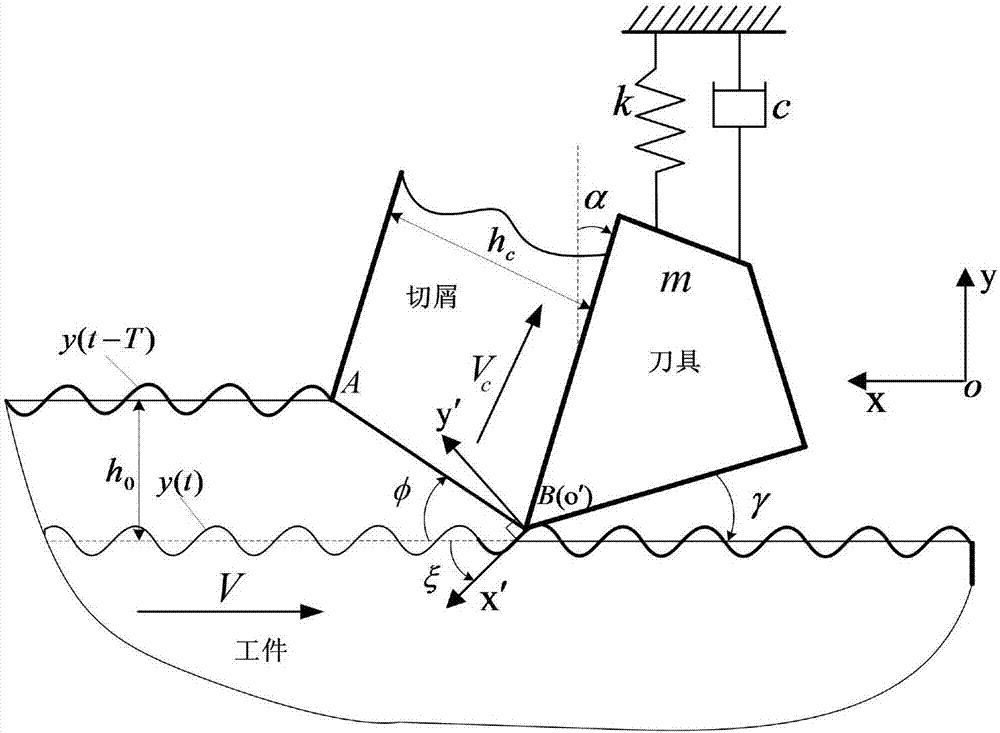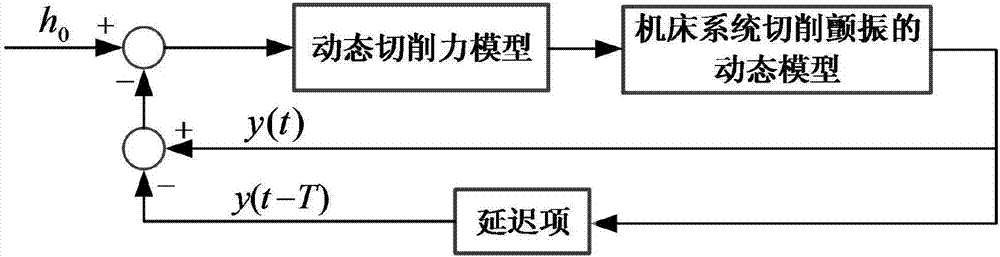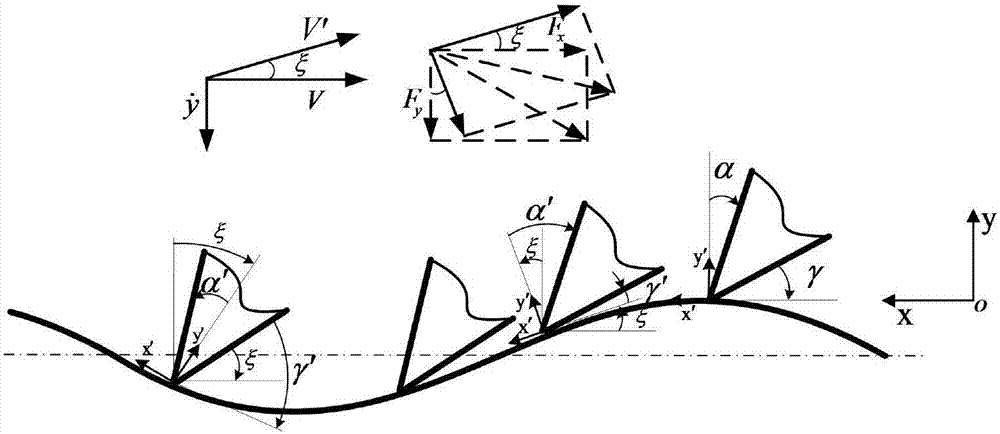# Right-angle cutting chatter analysis modeling method

## A technology of right-angle cutting and modeling method, which can be used in measuring/indicating equipment, program control, instruments, etc., and can solve problems such as cumbersomeness and low accuracy of cutting force coefficients

Active Publication Date: 2017-09-12
WUHAN INSTITUTE OF TECHNOLOGY
6 Cites 14 Cited by

## AI-Extracted Technical Summary

### Problems solved by technology

 In view of the present situation of the above-mentioned prior art, the technical problem to be solved by the present invention is to provide a right-angle cutting chatter analytical modeling method, which can overcome the problem that the accuracy of the cutting f...
View more

## Abstract

The invention relates to a right-angle cutting chatter analysis modeling method. The right-angle cutting chatter analysis modeling method comprises the following steps that firstly, a right-angle cutting chatter kinetic model is established; secondly, dynamic cutting force and a cutting force coefficient are calculated; and thirdly, a stability SLD figure of the right-angle cutting process is calculated. According to the right-angle cutting chatter analysis modeling method, the dynamic cutting process is regarded as the quasi-static cutting process at every moment, workpiece material features, tool geometry and cutting parameters are used as input parameters, the dynamic cutting force can be calculated through the cutting parameters which can be equivalently transformed, the expression of the dynamic cutting force coefficient is then theoretical deduced, through algebraic equation arrangement, the dynamic cutting force coefficient is obtained, the tediousness of experimental calibration is avoided, and the accuracy is improved; and in addition, the dynamic model of cutting chatter of a machine tool system can be expressed with a delay differential equation, the right-angle cutting chatter stability SLD figure is obtained with a time domain semi-discrete method through cutting stability analysis, and relatively real chatter stability prediction is provided.

Application Domain

Programme controlMeasurement/indication equipments

Technology Topic

Cutting forceMachine tool +6

## Image

•••## Examples

• Experimental program(1)

### Example Embodiment

 The present invention will be further described in detail with reference to the accompanying drawings and embodiments. The following embodiments are for explaining the present invention and the present invention is not limited to the following embodiments.
 Such as Figure 1~3 As shown, a right-angle cutting chatter analytical modeling method includes the following steps:
 Step 1. Establish a dynamic model of right-angle cutting chatter
 Determine the geometric parameters of the tool, the rake angle α, the unit is deg, the relief angle γ, the unit is deg; the selected cutting parameter, the cutting speed V, the unit is m/min, the feed amount f t , The unit is mm/r, the cutting width b, the unit is mm; in the metal cutting process, the change of the cutting thickness will cause the fluctuation of the cutting force, the cutting force and the cutting thickness change according to a specific cycle, and form a closed loop The feedback system calculates the dynamic cutting thickness h(t) through formula (1);
 h(t)=h 0 -[y(t)-y(t-T)] (1)
 Where h 0 Is the nominal cutting thickness, the unit is mm, and the value is equal to the tool feed f t; Y(t) and y(tT) respectively represent the vibration amplitude of the current and previous period in the y direction, the unit is mm, also known as internal modulation and external modulation, [y(t)-y(tT)] represents The cutting thickness difference due to tool vibration at time t, T is the delay between the current time and the previous time, that is, the spindle rotation period;
 When the workpiece is considered to be rigid and the tool is flexible, the dynamic model of the machine tool system's cutting chatter is a single-degree-of-freedom system in the feed direction, and the feed direction is the y direction. The vibration equation of the system is given by the formula (2 ) Means;

 Among them, m represents the equivalent mass of the system, the unit is kg, c represents the damping of the system, the unit is Ns/m, k represents the equivalent stiffness of the system, the unit is N/m, F y (t) is the dynamic cutting force caused by the dynamic cutting thickness h(t) in the feed direction;
 Step 2. Calculate dynamic cutting force and cutting force coefficient
 The dynamic cutting force is expressed by formula (3);
 F x (t)=k x (t)bh(t),F y (t)=k y (t)bh(t) (3)
 Where k x (t),k y (t) are the dynamic cutting force coefficients in the x and y directions, expressed as the nominal cutting speed V and the tool vibration speed The function of is represented by formula (4) and formula (5) respectively;


 Among them, O means high-order infinitesimal, c xi , C yi , I=0,1,2 is a function of the nominal cutting speed V, which is geometrically calibrated by cutting parameters within a given range;
 Regarding the chip formation process as a quasi-static process, the slope ξ of the cutting corrugated surface is the azimuth angle between the quasi-static direction and the effective instantaneous cutting direction, the quasi-static direction is the x direction, and the effective instantaneous cutting direction is x′ The direction, the slope ξ is expressed by formula (6);

 Among them, V is the nominal cutting speed in m/min, Is the tool vibration velocity in the y direction, in m/min, and the sign of ξ and The same;
 Then, the effective rake angle α'at time t, the unit is deg, the effective relief angle γ', the unit is deg,, the effective cutting thickness h'(t), the unit is mm, through the change between quasi-static cutting and dynamic cutting The relationship is obtained, which is represented by formula (7) and formula (8);
 α′=α-ξ, γ′=γ+ξ (7)

 Among them, the nominal rake angle α and the nominal clearance angle γ correspond to the rake angle and the relief angle of the quasi-static cutting process, in deg;
 Instantaneous shear angle φ′, effective cutting speed V′ and effective chip speed V′ c Calculated by formula (9) and formula (10) respectively;


 among them, Is the dynamic friction angle, the unit is deg. The dynamic friction angle is calculated by formula (11):

 among them, Is the nominal friction coefficient, p is the exponential parameter, V' c Is the effective chip speed, the unit is m/min;
 Through the conversion of the above formula, the final expression formula (12) of the instantaneous shear angle φ′ is obtained;

 Among them, A 1 , A 2 It is a material characteristic parameter, a dimensionless unit. The instantaneous shear angle φ′ depends on the nominal cutting speed V and the tool vibration speed Solved by Newton-Raphson iterative algorithm;
 Effective mean flow shear stress Determined by the Johnson-Cook constitutive model of the cutting material, the effective shear force F s ′, the cutting forces in the x and y directions are calculated by formula (13) and formula (14) respectively;


 In the quasi-static state at each moment, calculate the slope ξ of the cutting corrugated surface, effective rake angle α', effective relief angle γ'and effective cutting thickness h'(t), effective cutting speed V', and effective chip speed V' c , The instantaneous shear angle φ′, and then through the algebraic equation to get the dynamic cutting force coefficient k in the x and y directions x (t),k y (t);
 Step 3. Calculate the stability SLD diagram of the right-angle cutting process
 First, combine formula (2) and formula (5), and introduce a small amount of vibration u(t) at the equilibrium position to obtain the expression formula (15) of the dynamic model of the machine tool system cutting chatter vibration;

 Then by introducing a small amount of vibration at the equilibrium position shown in formula (15) By ignoring the influence of the nonlinear part at the right end of the formula (15), the linear analysis of the flutter is performed, and the formula (16) is obtained;

 make Organize and obtain the state space equation formula (17) of formula (15);

 among them,
 By numerically solving formula (17), the time period T is discretized into n equally divided cells, that is, T=nτ, in each interval [iτ,(i+1)τ](i=0,...,n -1) The intermediate matrix Φ by using continuous discrete mapping D i , The structure formula (18) is as follows;
 y n =Φy 0 =D n-1 D n-2 …D 1 D 0 y 0 (18)
 Among them, D i Is the discrete mapping matrix, y i Is a 2(n+1) column vector;
 Then according to the Floquet theory, that is, a linear ordinary differential equation with periodic coefficients, through reduction into a constant coefficient ordinary differential equation, the stability of the flutter system is judged. When the modulus of all eigenvalues ​​of the matrix Φ is less than unit 1, The system is stable, otherwise it is unstable, and then calculate the relationship between cutting speed and cutting width, which is the stability SLD diagram of the cutting chatter system.
 The analytical modeling process of the present invention will be explained more clearly in conjunction with a specific embodiment below.
 The flow shear stress of the workpiece material is calculated by the Johnson-Cook constitutive model. The dynamic model of the cutting chatter of the machine tool system during the right-angle cutting process is a single degree of freedom system in the feed direction. In addition, each cutting process uses a new blade , The parameters selected in this embodiment are shown in Table 1:
 Table 1 Parameters used in the analytical model

 Such as Figure 4 As shown, according to the same parameter data given in Table 1, the flutter SLD diagram is calculated by the proposed analytical model and the traditional flutter model. It can be seen that the results of these two models are very good in the high cutting speed area. However, the traditional chatter model is inconsistent with the experimental results and the finite element simulation results in the cutting speed area, and the analytical model proposed by the present invention has such low speed and high stability The phenomenon is due to the partial process damping introduced by the dynamic cutting force, which leads to an increase in flutter stability. Therefore, the analytical model proposed in the present invention clearly reflects this low-speed stability phenomenon and provides a more realistic flutter stability prediction , It can accurately predict the stability of the machining process, and solve the problem that the traditional chatter model cannot intuitively explain the thermal characteristics in the actual cutting process and the accuracy of the cutting force coefficients calibrated by experiments is too low.
 Obviously, those skilled in the art can make various modifications and variations to the present invention without departing from the spirit and scope of the present invention. If these modifications and variations of the present invention fall within the scope of the claims of the present invention and their equivalent technologies, the present invention The invention includes these changes and modifications, and the content not described in detail in this specification belongs to the prior art known to those skilled in the art.

## PUM## Description & Claims & Application Information

We can also present the details of the Description, Claims and Application information to help users get a comprehensive understanding of the technical details of the patent, such as background art, summary of invention, brief description of drawings, description of embodiments, and other original content. On the other hand, users can also determine the specific scope of protection of the technology through the list of claims; as well as understand the changes in the life cycle of the technology with the presentation of the patent timeline. Login to view more.
Who we serve
• R&D Engineer
• R&D Manager
• IP Professional
Why Eureka